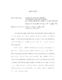## Applications of Physics to Finance and Economics: Returns, Trading Activity and Income##### Files
umi-umd-2486.pdf(10.81 MB)
This dissertation reports work where physics methods are applied to financial and economical problems. Some material in this thesis is based on $3$ published papers \cite{SY,SPY,income} which divide this study into two parts. The first part studies stock market data (chapter 1 to 5). The second part is devoted to personal income in the USA (chapter 6). We first study the probability distribution of stock returns at mesoscopic time lags (return horizons) ranging from about an hour to about a month. While at shorter microscopic time lags the distribution has power-law tails, for mesoscopic times the bulk of the distribution (more than 99\% of the probability) follows an exponential law. The slope of the exponential function is determined by the variance of returns, which increases proportionally to the time lag. At longer times, the exponential law continuously evolves into Gaussian distribution. The exponential-to-Gaussian crossover is well described by the analytical solution of the Heston model with stochastic volatility. After characterizing the stock returns at mesoscopic time lags, we study the subordination hypothesis with one year of intraday data. We verify that the integrated volatility $V_t$ constructed from the number of trades process can be used as a subordinator for a driftless Brownian motion. This subordination will be able to describe $\approx 85\%$ of the stock returns for intraday time lags that start at $\approx 1$ hour but are shorter than one day (upper time limit is restricted by the short data span of one year). We also show that the Heston model can be constructed by subordinating a Brownian motion with the CIR process. Finally, we show that the CIR process describes well enough the empirical $V_t$ process, such that the corresponding Heston model is able to describe the log-returns $x_t$ process, with approximately the maximum quality that the subordination allows ($80\% - 85\%$). Finally, we study the time evolution of the personal income distribution. We find that the personal income distribution in the USA has a well-defined two-income-class structure. The majority of population (97--99\%) belongs to the lower income class characterized by the exponential Boltzmann-Gibbs (thermal'') distribution, whereas the higher income class (1--3\% of population) has a Pareto power-law (superthermal'') distribution. By analyzing income data for 1983--2001, we show that the thermal'' part is stationary in time, save for a gradual increase of the effective temperature, whereas the superthermal'' tail swells and shrinks following the stock market. We discuss the concept of equilibrium inequality in a society, based on the principle of maximal entropy, and quantitatively show that it applies to the majority of population.